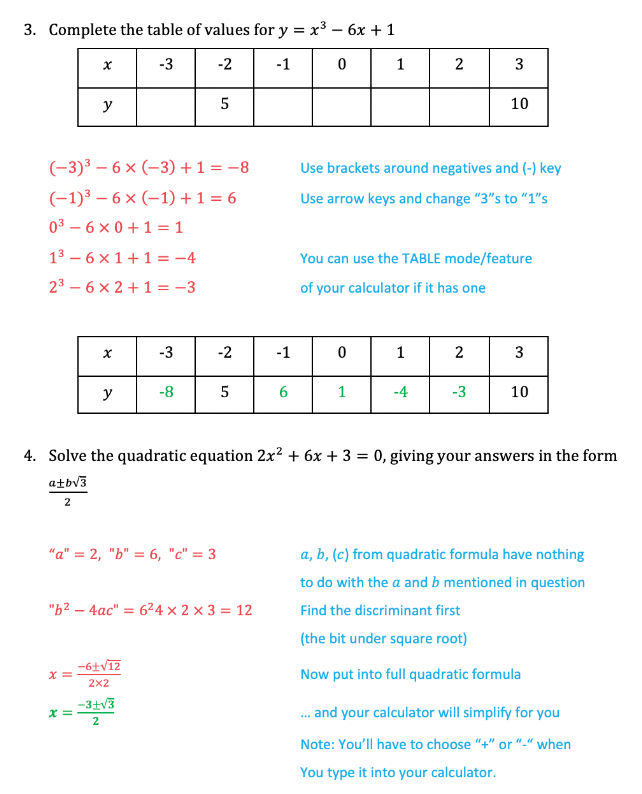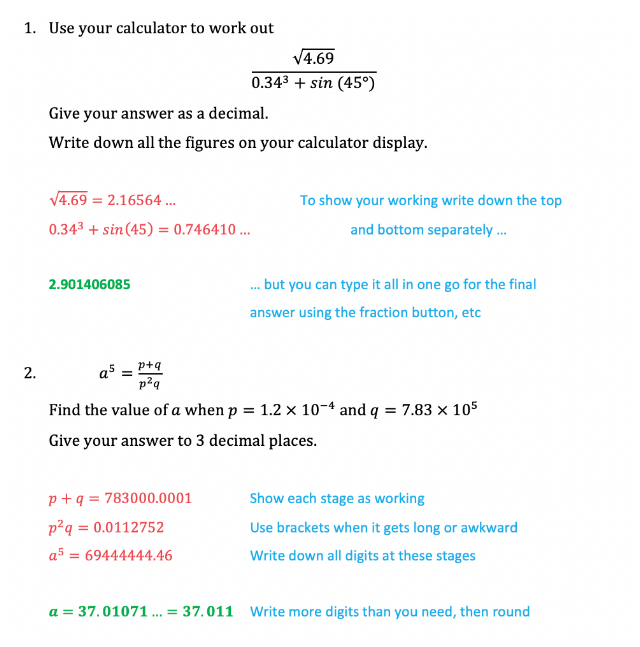# Edexcel IGCSE Maths 复习笔记 1.16.1 Using a Calculator

Edexcel IGCSE Maths 复习笔记 1.16.1 Using a Calculator

#### Why the fuss about using a calculator?

• GCSE Mathematics goes beyond using the basic features of a calculator and explores many of the special functions of a scientific calculator
• It is important to get to know your calculator, the earlier you get one and learn about the scientific functions the better you will be at using them
• It’s not just maths that uses these, some of the scientific functions can be used in science exams too

#### What do I need to know?

• The notes below apply to most if not all scientific calculators but the images are based on the Casio fx-83GTX
• The Casio fx-85GTX is the same model but also has solar power. Both are labelled “Classwiz” too but be careful here at there is a more advanced “Classwiz” calculator that is used at A level (fx-991EX)• Be aware if you have an old or very basic scientific calculator that they may work backwards
• For example, if you wanted to find sin (57) you would type 57 then press the sin button
• Modern calculators tend to work in the order in which we write things

1. Mode/setup

• Make sure you know how to change the mode of your calculator, especially if someone else has used it
• The “Angle Unit” needs to be degrees – normally indicated by a “D” symbol across the top of the display
• Make sure you can switch between “exact” answers (fractions, surds, in terms of π, etc) and “approximate” answers (decimals)
• Most calculators default to “Math” mode with the word Math written across the top of the display or using a symbol
• When in “Math” mode you can switch whatever is on the answer line between exact and decimals by pressing the “S-D” button

2. Templates

• These are largely the shortcut buttons – the fraction button, the square, cube and power buttons, square roots3. Trigonometry (sin/cos/tan)

• Remember to use SHIFT (sometimes called 2nd or INV button) when finding angles
• When using these buttons you will find that before you type the angle the calculator automatically gives you an open bracket "(". You should get into the habit of making sure you use a closed bracket ")" after typing the angle in
• This is very important if there is something else to type in that comes after sin/cos/tan

4. Standard Form and π

• Find the ×10x button and know how to use it
• Modern calculators display standard form in the way it is written
• Older models may use a small capital letter "E" in place of ×10on the display line
• π is often near or under SHIFT with the standard form button

5. Memory

• The ANS (answer) button is very useful – especially when working with decimals in the middle of solutions that you should avoid rounding until your final answer
• ANS recalls the last answer the calculator calculated

6.Table

• If your calculator has a table function or mode, use it
• This can be extremely useful in those “complete the table of values and draw the graph” type questions

7. Brackets and negative numbers

• Use as you would in written mathematics
• Remember to use the (-) button for a negative number, not the subtract button

8. Judgement and special features

• The rule of thumb is to use your calculator to do one calculation at a time
• However, you can also make a judgement call on this as to how many marks are available in the question and whether a question asks you to “write down all the digits on your calculator display”
• You are better off writing too much down than not enough!

9. Practise!

• This is a long list but we will finish by going back to the start – there is nothing better you can do than getting a calculator early and learning how to use it by practising the varying types of questions you are likely to come across

#### Exam Tip

Always put negative numbers in brackets. For a quick example, try using your calculator to work out -32 and then (-3)2.In working out always write down more digits than the final answer requires and don’t round them (write something like 9.3564… using the three dots shows you haven’t rounded).  Use the ANS button when you next need that number on your calculator.

#### Worked Example